# Kruskal’s algorithm In Python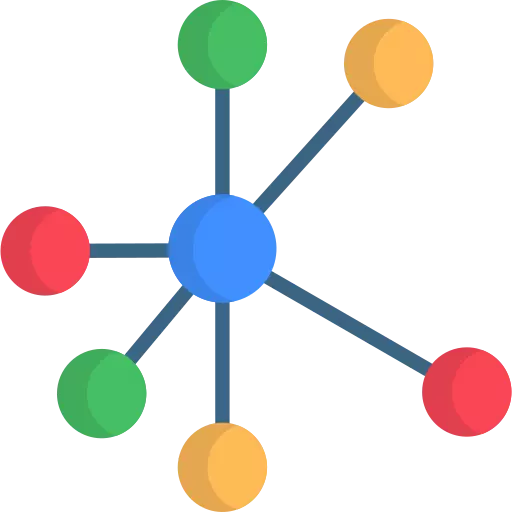## Kruskal’s algorithm Implementation in Python

Kruskal’s algorithm, a fundamental concept in graph theory, provides a straightforward approach to find the minimum spanning tree of a connected graph. This tree ensures the most efficient connection between all the vertices while minimizing the total edge weight.

In this tutorial, we’ll simplify the complexities of Kruskal’s algorithm and guide you through its implementation in Python.

## What is Kruskal’s Algorithm?

Kruskal’s algorithm is a greedy algorithm used to find the minimum spanning tree of a connected, undirected graph. In simpler terms, it helps to find the most efficient way to connect all the vertices in a graph while minimizing the total edge weight.

### Step by Step working of kruskal’s algorithm :

Below is the illustration of the kruskal’s algorithm :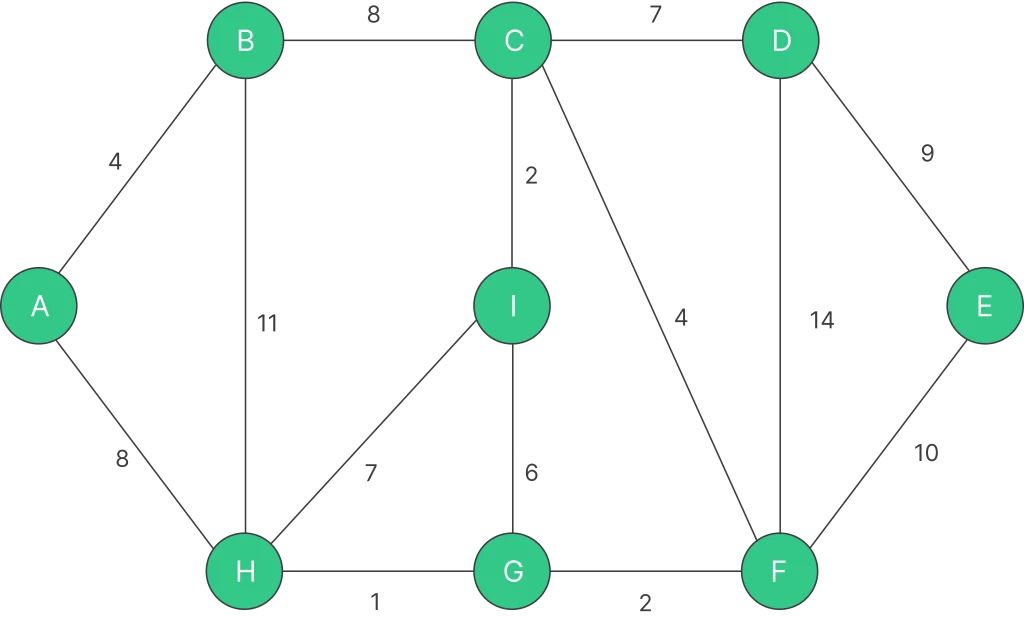The graph consists of 9 vertices and 14 edges. Therefore, the minimum spanning tree formed will comprise 8 edges, as it connects all 9 vertices with the minimum total weight.

After sorting:

Weight SourceDestination
1HG
2IC
2GF
4AB
4CF
6IG
7CD
7GI
8AH
8BC
9DE
10FE
11BH
14DF

Now pick all edges one by one from the sorted list of edges.

Step 1: Pick edge H – G. No cycle is formed, include it.Step 2:  Pick edge I – C. No cycle is formed, include it.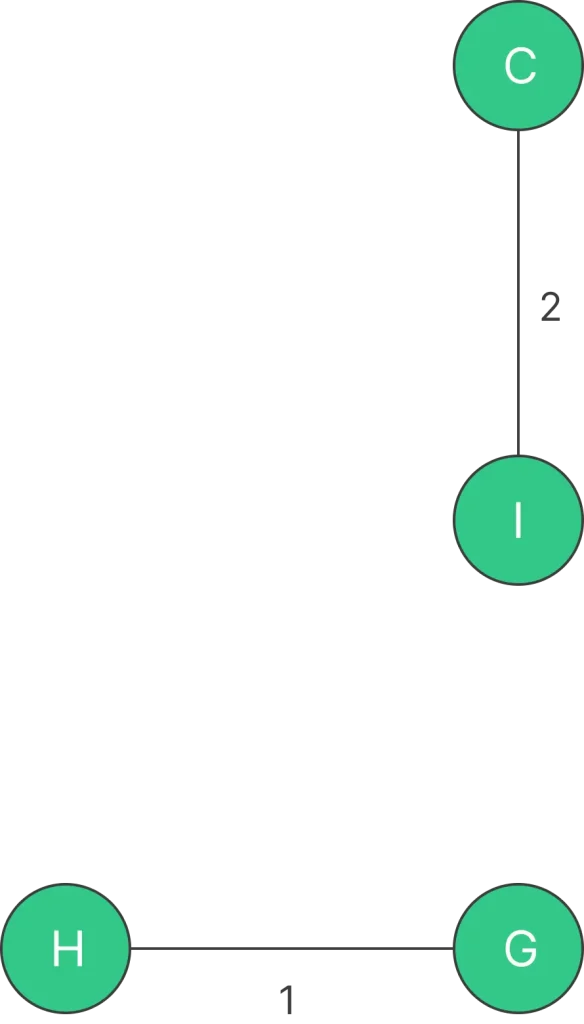Step 3: Pick edge G – F. No cycle is formed, include it.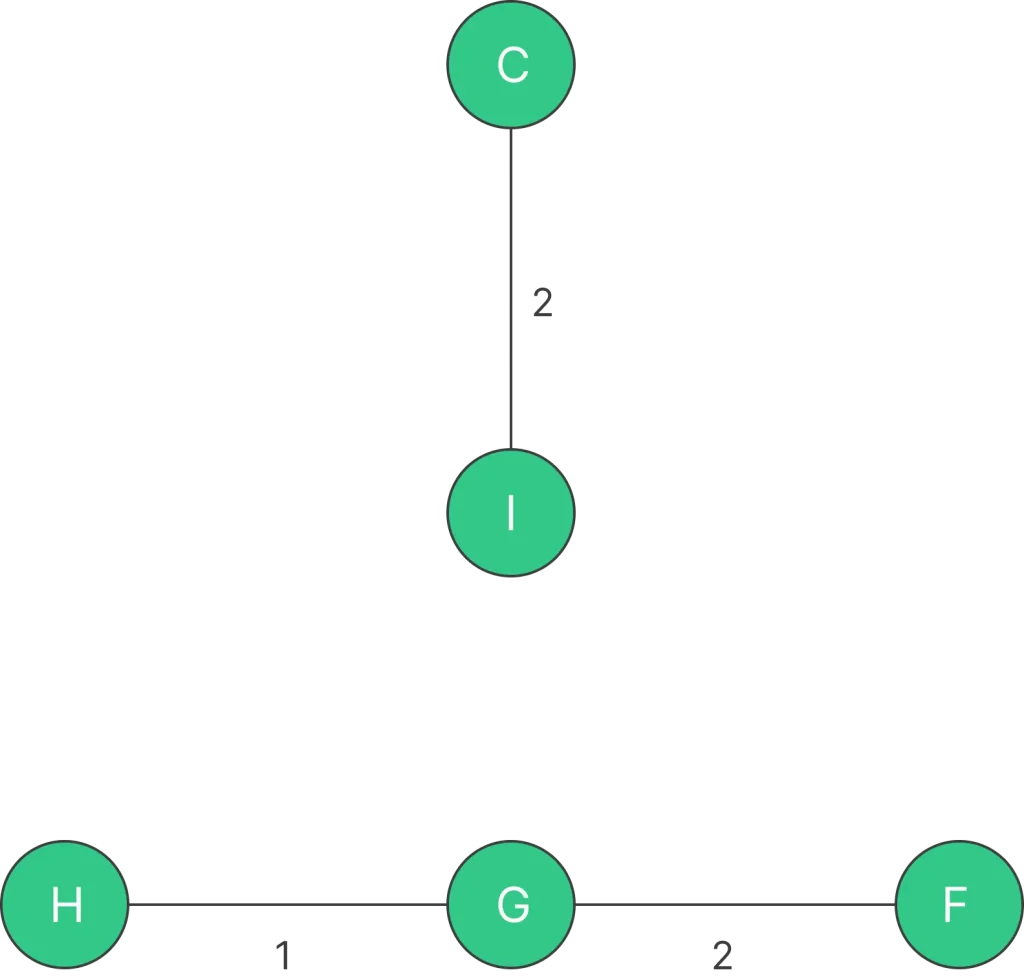Step 4: Pick edge A – B. No cycle is formed, include it.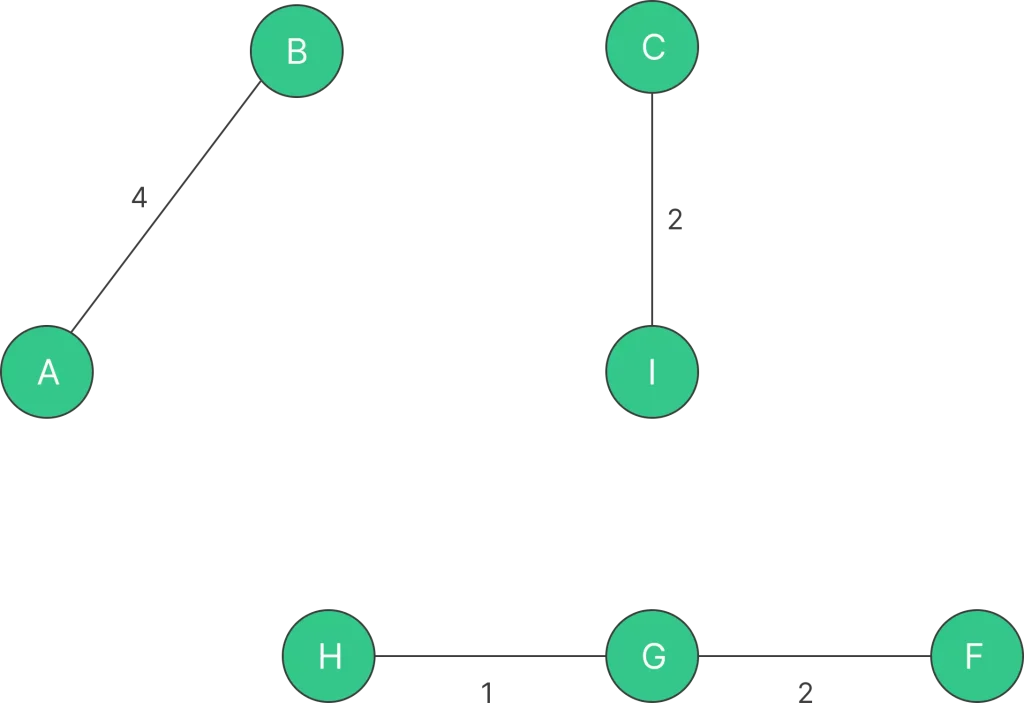Step 5: Pick edge C – F. No cycle is formed, include it.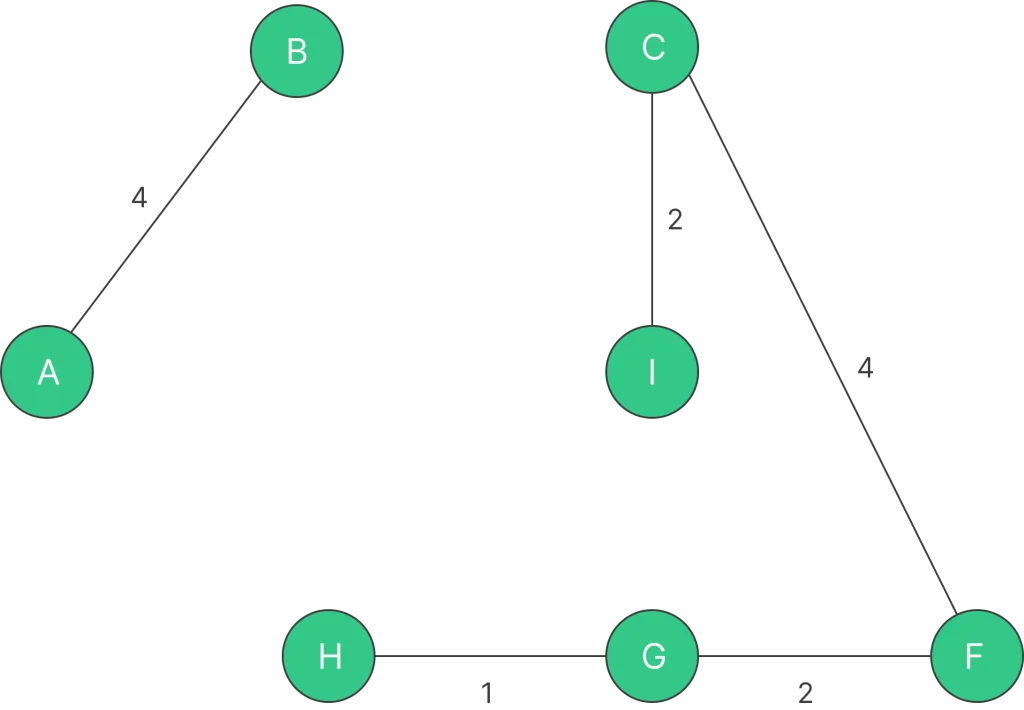Step 6: Pick edge I – G. Since including this edge results in the cycle, discard it. Pick edge C – D: No cycle is formed, include it.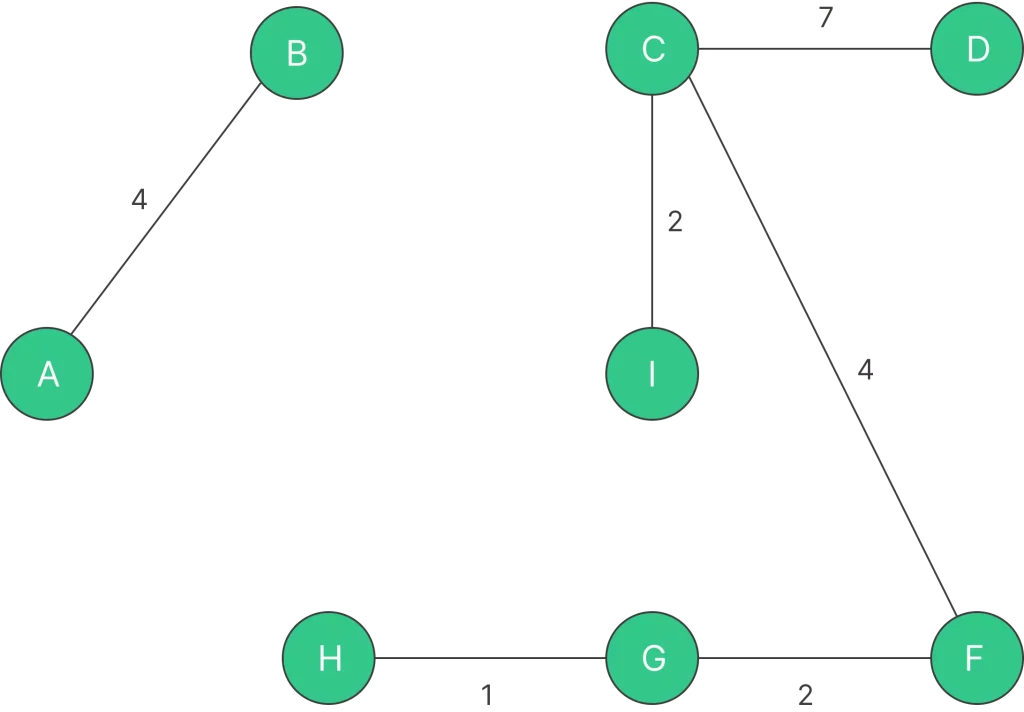Step 7: Pick edge H – I. Since including this edge results in the cycle, discard it. Pick edge A – H. No cycle is formed, include it.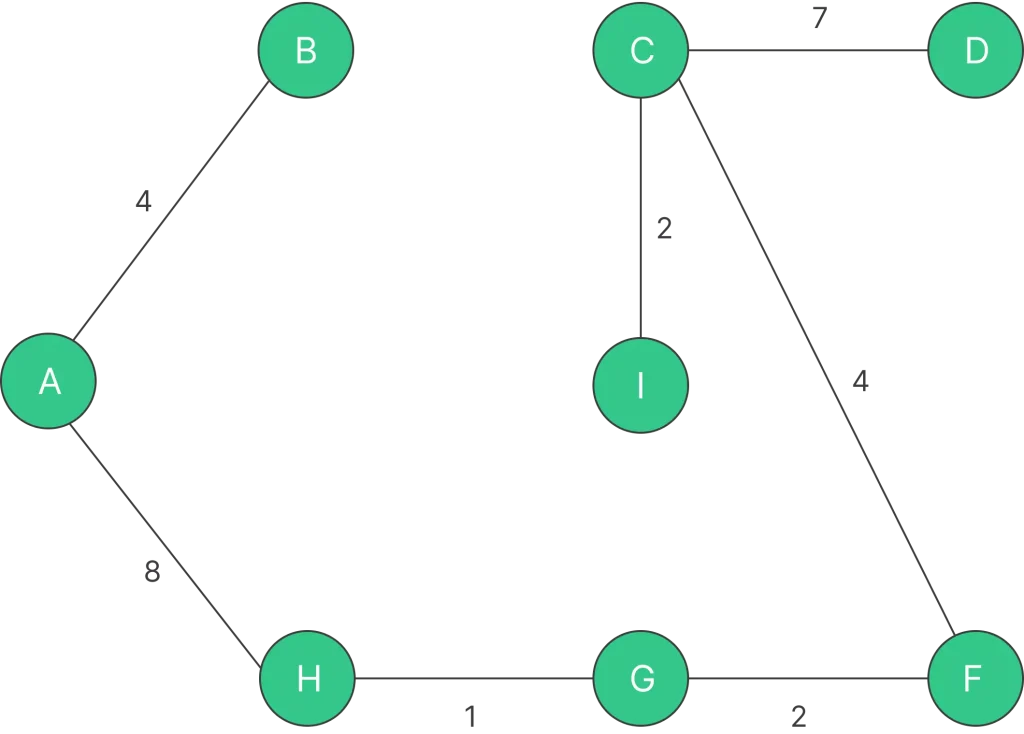Step 8: Pick edge B – C. Since including this edge results in the cycle, discard it. Pick edge D – E. No cycle is formed, include it.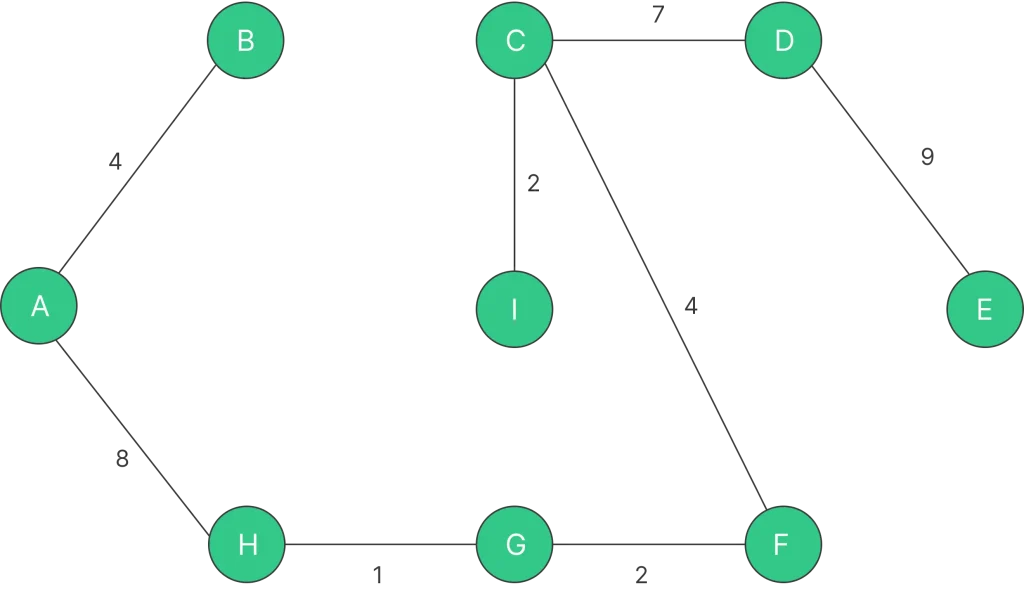### Kruskal’s Algorithm Pseudocode :

```Kruskal's Algorithm:
1. Initialize an empty list called "MST" to store the edges of the minimum spanning tree.
2. Sort all the edges of the graph in non-decreasing order of their weights.
3. Initialize a disjoint set data structure to keep track of the connected components of the graph.
4. For each edge (u, v) in the sorted list of edges:
a. If adding the edge (u, v) to the MST does not create a cycle in the MST:
i. Add the edge (u, v) to the MST.
ii. Merge the connected components of u and v in the disjoint set data structure.
5. Repeat step 4 until MST contains (V - 1) edges, where V is the number of vertices in the graph.
6. MST now contains the edges of the minimum spanning tree.

Disjoint Set Data Structure Operations:
- MakeSet(v): Create a new set with a single element v.
- FindSet(v): Find the representative of the set containing v.
- UnionSets(u, v): Merge the sets containing u and v into a single set.

Note: The pseudocode above assumes that the graph is represented as a list of edges, where each edge has a source vertex, a destination vertex, and a weight.
```

### Python Implementation for Kruskal’s Algorithm :

```class DisjointSet:
def __init__(self, vertices):
self.parent = [i for i in range(vertices)]
self.rank =  * vertices

def find(self, node):
if self.parent[node] != node:
self.parent[node] = self.find(self.parent[node])
return self.parent[node]

def union(self, u, v):
rootU = self.find(u)
rootV = self.find(v)

if self.rank[rootU] > self.rank[rootV]:
self.parent[rootV] = rootU
elif self.rank[rootU] < self.rank[rootV]:
self.parent[rootU] = rootV
else:
self.parent[rootV] = rootU
self.rank[rootU] += 1

class Graph:
def __init__(self, vertices):
self.V = vertices
self.graph = []

self.graph.append([u, v, weight])

def kruskal(self):
self.graph = sorted(self.graph, key=lambda item: item)
mst = []
ds = DisjointSet(self.V)

for edge in self.graph:
u, v, weight = edge
if ds.find(u) != ds.find(v):
mst.append([u, v, weight])
ds.union(u, v)

return mst

# Example usage
g = Graph(4)

minimum_spanning_tree = g.kruskal()
print("Edges in the Minimum Spanning Tree:")
for edge in minimum_spanning_tree:
print(edge, "-", edge, ":", edge)
```

### Time and Space Complexity for Kruskal’s Algorithm :

Time Complexity:

• Sorting the edges: O(E log E), where E is the number of edges in the graph. Sorting the edges is the most time-consuming step in Kruskal’s algorithm.
• Union-Find Operations: O(E log V), where V is the number of vertices in the graph. Kruskal’s algorithm processes edges in ascending order of weight and performs union-find operations on the vertices of each edge to check for cycles. The union-find operations take O(log V) time in the worst case. Since there are E edges, the total time complexity for union-find operations is O(E log V).

Space Complexity:

• The space complexity of the adjacency list representation of the graph is O(V + E), where V is the number of vertices and E is the number of edges.
• The space complexity of the disjoint-set data structure (union-find) is O(V) since it needs to store information for each vertex.

Therefore, the overall space complexity of Kruskal’s algorithm is O(V + E).

### Related Banners

Get PrepInsta Prime & get Access to all 200+ courses offered by PrepInsta in One Subscription

## Get over 200+ course One Subscription

Courses like AI/ML, Cloud Computing, Ethical Hacking, C, C++, Java, Python, DSA (All Languages), Competitive Coding (All Languages), TCS, Infosys, Wipro, Amazon, DBMS, SQL and others